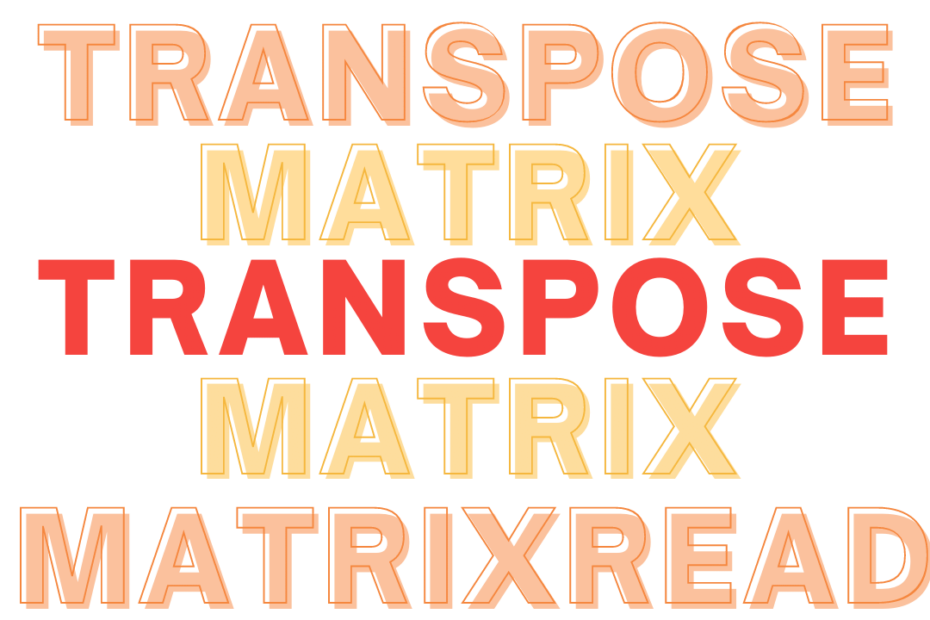# C++## Transpose a Matrix

• DSA

Given a matrix of N dimensions, and we need to transpose it. One of the standard problem on matrices. Don’t confuse Transpose with Rotation, the rotation is normally performed on the X-Y axis while in a transpose, the matrix is flipped on its diagonal. Example: Solution Approach This is simple… Read More »Transpose a Matrix## How the bits/stdc++ .h works in C++

• DSA

I often wonder, How does #include < bits/stdc++.h> work?. I’ve seen this while solving competitive problems and referring to various solutions and I wanted to know what’s happening in the background. Here’s what I found It is a precompiled header file that contains every standard library Once, we use this… Read More »How the bits/stdc++ .h works in C++## Perform Set Operations on Arrays

• DSA

In this problem, we’ll be performing some basic set operations on the given arrays like Union, Intersection, and Difference. I found this problem when I was writing a practice test on ADT (Array as a Data Structure). Examples Set Operations Union ∪ A union of two sets will contain the… Read More »Perform Set Operations on Arrays## Target Sum – Single Iteration O(N)

• DSA

Given, a sorted array of elements and we need to find all the pairs of elements that add up equally to a given target sum number, this can be done usually done using two loops, like a brute force method where all the elements are compared with every other element… Read More »Target Sum – Single Iteration O(N)## Sum of Numbers in a String

• DSA

Today, I found a problem where we need to find the sum of numbers in a string, the string will be a mix of alphabets, numbers, and special characters, and usually, this can be solved by iterating through each character and adding all the numbers. Example Solution Approach Given a… Read More »Sum of Numbers in a String## Squares of a Sorted Array LeetCode O(N)

• DSA

In this problem, given a sorted array in decreasing order and we should return the squares of each number and in the same ascending order, and the one thing we need to take care of is that the negative numbers, which when squared disturb the ascending ordered array. Problem Statement… Read More »Squares of a Sorted Array LeetCode O(N)